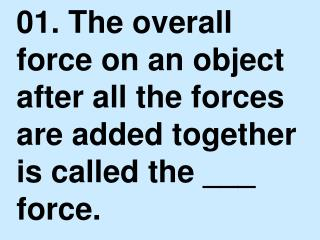DownloadDownload PresentationNET

# NET

Télécharger la présentation## NET

- - - - - - - - - - - - - - - - - - - - - - - - - - - E N D - - - - - - - - - - - - - - - - - - - - - - - - - - -
##### Presentation Transcript

1. 01. The overall force on an object after all the forces are added together is called the ___ force.

2. NET

3. ACCELERATED

4. INERTIA

5. 04. One ___ is the force required to accelerate 1 kilogram of mass at 1 meter per second per second.

6. NEWTON

7. 05. A person traveling in a car that stops suddenly keeps moving forward because of ___.

8. INERTIA

9. 06. Friction acts in a direction ___ to an object’s direction of motion.

10. OPPOSITE

11. 07. Wet pavement is more slippery than dry pavement because the force needed to overcome ___ friction is less than the force needed to overcome sliding friction.

12. FLUID

13. 08. The downward force acting on an object in free fall is the force of ___.

14. GRAVITY

15. 09. A(n) ___ is an object that is thrown.

16. PROJECTILE

17. 10. When ___ equals the force of gravity on a falling object, the object reaches terminal velocity.

18. AIR RESISTANCE

19. 11. The metric unit that is most often used to describe weight is the ___.

20. NEWTON

21. 12. The force of gravity between you and Earth is greater than the force of gravity between you and a car because Earth has more ___ than the car.

22. MASS

23. 13. As the distance between two objects decreases, the gravitational force between them ___.

24. INCREASES

25. 14. According to Newton’s third law of motion, the strength of a reaction force is ___ the strength of the action force.

26. EQUAL TO

27. 15. If the action force of a bat striking a ball accelerates the ball in one direction, the reaction force accelerates the bat in the ___ direction.

28. OPPOSITE

29. DIRECTION

30. 17. The momentum of a skate boarder is not conserved because ___ acts on the wheels.

31. FRICTION

32. 18. During lift-off, the thrust on a rocket is directed ___.

33. UPWARD

34. 19. The moon is a natural ___ of Earth.

35. SATELLITE

36. 20. The force of gravity is responsible for continuously changing the ___ in which a satellite moves.

37. DIRECTION

38. FORCE

39. 22. When two equal forces act on the same object in opposite directions, the net force is ___.

40. ZERO

41. 23. When two forces act in the same direction, they ___.

42. COMBINE

43. INERTIA

44. 25. The amount of matter in an object is called its ___.

45. MASS